# Contravariance

Episode #14 • Apr 30, 2018 • Subscriber-Only

Let’s explore a type of composition that defies our intuitions. It appears to go in the opposite direction than we are used to. We’ll show that this composition is completely natural, hiding right in plain sight, and in fact related to the Liskov Substitution Principle.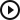Contravariance
Introduction
00:05
Variance in subtyping
01:05
Variance in functional programming
10:13
Positive and negative positions
13:45
What’s the point?
19:51
PredicateSet
22:22
PredicateSet and contramap
33:28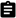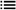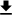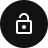### Introduction

Last week we took a deep dive into the `map` function. First we saw that `map` on arrays is unique amongst all the functions that transform arrays and satisfy a simple property. That was kind of amazing, because it shows that `map` wasn’t invented because it was convenient, rather it was only sitting there waiting for us to discover it.

In today’s episode we want to explore the idea of “contravariance”. It’s a kind of composition that hides right in plain sight in the programming world, but for whatever reason we never really come face-to-face with it. So we want to dedicate some time to really study it and build intuitions for it, because it will allow us to uncover new ways of composing things that are otherwise difficult to see.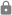This episode is for subscribers only.

### Subscribe to Point-Free

Access this episode, plus all past and future episodes when you become a subscriber.

See plans and pricing

### Exercises

1. Determine the sign of all the type parameters in the function `(A) -> (B) -> C`. Note that this is a curried function. It may be helpful to fully parenthesize the expression before determining variance.

Solution

Recall from the episode on exponents and algebraic data types that function arrows parenthesize to the right, which means when we write `(A) -> (B) -> C`, we really mean `(A) -> ((B) -> C)`. Now we can apply the bracketing method we demonstrated in the episode:

``````// (A) -> ((B) -> C)
//         |_|   |_|
//         -1    +1
// |_|    |________|
// -1        +1
``````

So now we see that `A` is negative, `B` is also negative, and `C` is positive.

2. Determine the sign of all the type parameters in the following function:

``````(A, B) -> (((C) -> (D) -> E) -> F) -> G
``````
Solution

We will apply the bracketing method to this expression, it’s just a little more involved:

``````// (A, B) -> (((C) -> (D) -> E) -> F) -> G
//             |_|    |_|   |_|
//             -1     -1    +1
// |_||_|     |_______________|   |_|
// +1 +1             -1           +1
// |____|    |______________________|   |_|
//   -1                 -1              +1
``````

That’s intense! One tricky part is how we determined that the `A` and `B` inside the tuple were in positive position, and this is because tuples are naturally a covariant structure: you can define `map` on the first and second components.

Now all we have to do is trace through the layers and multiply all the signs:

``````* A = -1 * +1 = -1
* B = -1 * +1 = -1
* C = -1 * -1 * -1 = -1
* D = -1 * -1 * -1 = -1
* E = -1 * -1 * +1 = +1
* F = -1 * +1 = -1
* G = +1
``````

And there you have it!

3. Recall that a setter is just a function `((A) -> B) -> (S) -> T`. Determine the variance of each type parameter, and define a `map` and `contramap` for each one. Further, for each `map` and `contramap` write a description of what those operations mean intuitively in terms of setters.

Solution

Again applying the bracketing method we see:

``````// ((A) -> B) -> (S) -> T
//  |_|   |_|
//  -1    +1
// |________|    |_|   |_|
//     -1        -1    +1
``````

So now we see:

• `A = -1 * -1 = +1`
• `B = -1 * +1 = -1`
• `S = -1`
• `T = +1`

This means we should be able to define `map` on `A` and `T`, and `contramap` on `B` and `S`. Here are the implementations of each, with comments that show the types of all the parts we need to plug together:

``````typealias Setter<S, T, A, B> = ((@escaping (A) -> B) -> (S) -> T

func map<S, T, A, B, C>(_ f: @escaping (A) -> C)
-> (@escaping Setter<S, T, A, B>)
-> Setter<S, T, C, B> {

return { setter in
return { update in
return { s in
// f: (A) -> C
// setter: ((A) -> B) -> (S) -> T
// update: (C) -> B
// s: S
setter(f >>> update)(s)
}
}
}
}

func map<S, T, U, A, B>(_ f: @escaping (T) -> U)
-> (@escaping Setter<S, T, A, B>)
-> Setter<S, U, A, B> {

return { setter in
return { update in
return { s in
// f: (T) -> U
// setter: ((A) -> B) -> (S) -> T
// update: (A) -> B
// s: S
f(setter(update)(s))
}
}
}
}

func contramap<S, T, A, B, C>(_ f: @escaping (C) -> B)
-> (@escaping Setter<S, T, A, B>)
-> Setter<S, T, A, C> {

return { setter in
return { update in
return { s in
// f: (C) -> B
// setter: ((A) -> B) -> (S) -> T
// update: (A) -> C
// s: S
setter(update >>> f)(s)
}
}
}
}

func contramap<S, T, U, A, B>(_ f: @escaping (U) -> S)
-> (@escaping Setter<S, T, A, B>)
-> Setter<U, T, A, B> {

return { setter in
return { update in
return { u in
// f: (U) -> S
// setter: ((A) -> B) -> (S) -> T
// update: (A) -> B
// u: U
setter(update)(f(u))
}
}
}
}
``````

It’s interesting to see that the implementation of `map` on `A` is quite similar to `contramap` on `B`, and `map` on `T` is similar to `contramap` on `S`.

4. Define `union`, `intersect`, and `invert` on `PredicateSet`.

5. This collection of exercises explores building up complex predicate sets and understanding their performance characteristics.

1. Create a predicate set `powersOf2: PredicateSet<Int>` that determines if a value is a power of `2`, i.e. `2^n` for some `n: Int`.
2. Use the above predicate set to derive a new one `powersOf2Minus1: PredicateSet<Int>` that tests if a number is of the form `2^n - 1` for `n: Int`.
3. Find an algorithm online for testing if an integer is prime, and turn it into a predicate `primes: PredicateSet<Int>`.
4. The intersection `primes.intersect(powersOf2Minus1)` consists of numbers known as Mersenne primes. Compute the first 10.
5. Recall that `&&` and `||` are short-circuiting in Swift. How does that translate to `union` and `intersect`?
6. What is the difference between `primes.intersect(powersOf2Minus1)` and `powersOf2Minus1.intersect(primes)`? Which one represents a more performant predicate set?
6. It turns out that dictionaries `[K: V]` do not have `map` on `K` for all the same reasons `Set` does not. There is an alternative way to define dictionaries in terms of functions. Do that and define `map` and `contramap` on that new structure.

7. Define `CharacterSet` as a type alias of `PredicateSet`, and construct some of the sets that are currently available in the API.

8. Let’s explore what happens when a type parameter appears multiple times in a function signature.

1. Is `A` in positive or negative position in the function `(B) -> (A, A)`? Define either `map` or `contramap` on `A`.
2. Is `A` in positive or negative position in `(A, A) -> B`? Define either `map` or `contramap`.
3. Consider the type `struct Endo<A> { let apply: (A) -> A }`. This type is called `Endo` because functions whose input type is the same as the output type are called “endomorphisms”. Notice that `A` is in both positive and negative position. Does that mean that both `map` and `contramap` can be defined, or that neither can be defined?
4. Turns out, `Endo` has a different structure on it known as an “invariant structure”, and it comes equipped with a different kind of function called `imap`. Can you figure out what it’s signature should be?
9. Consider the type `struct Equate<A> { let equals: (A, A) -> Bool }`. This is just a struct wrapper around an equality check. You can think of it as a kind of “type erased” `Equatable` protocol. Write `contramap` for this type.

10. Consider the value `intEquate = Equate<Int> { \$0 == \$1 }`. Continuing the “type erased” analogy, this is like a “witness” to the `Equatable` conformance of `Int`. Show how to use `contramap` defined above to transform `intEquate` into something that defines equality of strings based on their character count.